Scale
Objectives

To calculate scale in three different ways (i.e. linear, representative fraction, and verbal scale). To move from one type of scale to another. To measure distances along curved and straights lines. To introduce the concepts of magnetic declination, bearings, and azimuths for potential use in future geography courses in college or university. (Note: Magnetic Declination, Bearings, and Azimuths will not be tested in Geography 12).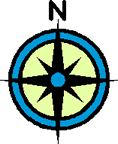Let's look at the concept of map scale and how to measure distances along straight and curved lines.

 Scale Notes - Download, Print Out, and Read Carefully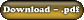Example A) Convert the following representative fraction into a verbal scale. 1) 1:25 000 Answer: 1:25 000 means that 1cm on the map represents 25 000 cm on the ground in reality. However, when talking about distances on the ground, we normally think in terms of kilometres. Recall, that there are 100 000 cm in 1 km. Therefore, 25 000 / 100 000 = 0.25 km. Verbal Scale: 1 cm represents 0.25 km B) Convert the following verbal scale into a representative fraction. 1) 1 cm represents 0.75 km Answer: Convert 0.75 km into centimetres by multiplying by 100 000. Therefore, 0.75 x 100 000 = 75 000. Representative Fraction: 1:75 000 C) Convert the following into a linear scale. 1) 1 cm represents 2.5 km. Answer: Draw a line with one centimetre intervals. Each interval represents a distance of 2.5 km. Remember to label the kilometres. Linear Scale: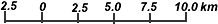D) Find the actual distance along the road from Town A to Town B on the map below.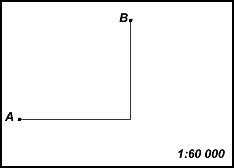Answer: Measure the first segment along the road from A and then the remaining segment to B. Note: Measure to one decimal place accuracy. i.e.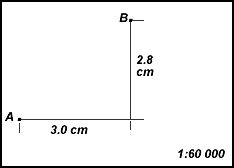Therefore, the total map distance along the road from A to B is 5.8 cm. Now, apply the scale. 1:60 000 means that every centimetre on the map represents 0.6 km in reality. Hence, the actual distance between Town A and Town B is: 5.8 cm x 0.6 km = 3.48 km. E) Find the distance along the curved road from Town A to Town B on the map below.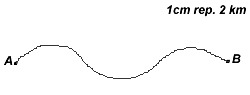Answer: To find the map distance between A and B below, one could use a string, however you cannot use string on the government exam. Therefore, another technique is the paper edge method. Here, you start by putting the edge of a piece of paper next to A and marking A on the edge of the paper. Then put the edge of the paper as far along the road until the road curves away. Put a mark where it curves away (see diagram below).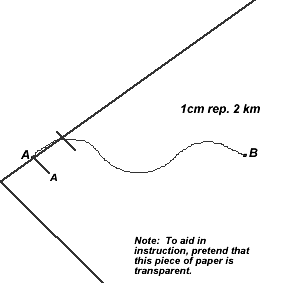Next, pivot your paper along the last tick mark to expose another section of straight road. Put a tick mark again where the road begins to curve away (see diagram below).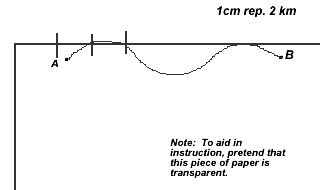Pivot to another straight section of road and make a tick mark again where the road curves away (see diagram below).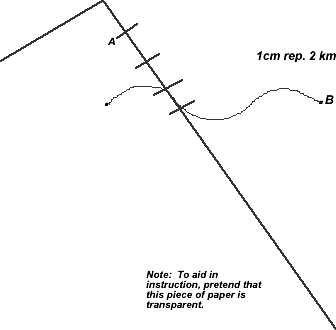Continue in a similar fashion until you reach B. Then take your ruler and measure the distance between A and B on your paper to one decimal place accuracy. In this example, the distance is approximately 6.4cm. Then apply the scale. 6.4 cm x 2 km = 12.8 km which is the actual distance between Town A and Town B.

 Assignment Work 1) Download, print out, and complete the Scale Exercises.2) When done, check over your answers using the key.©www.coolschool.ca Press the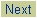button on the Action menu to proceed.